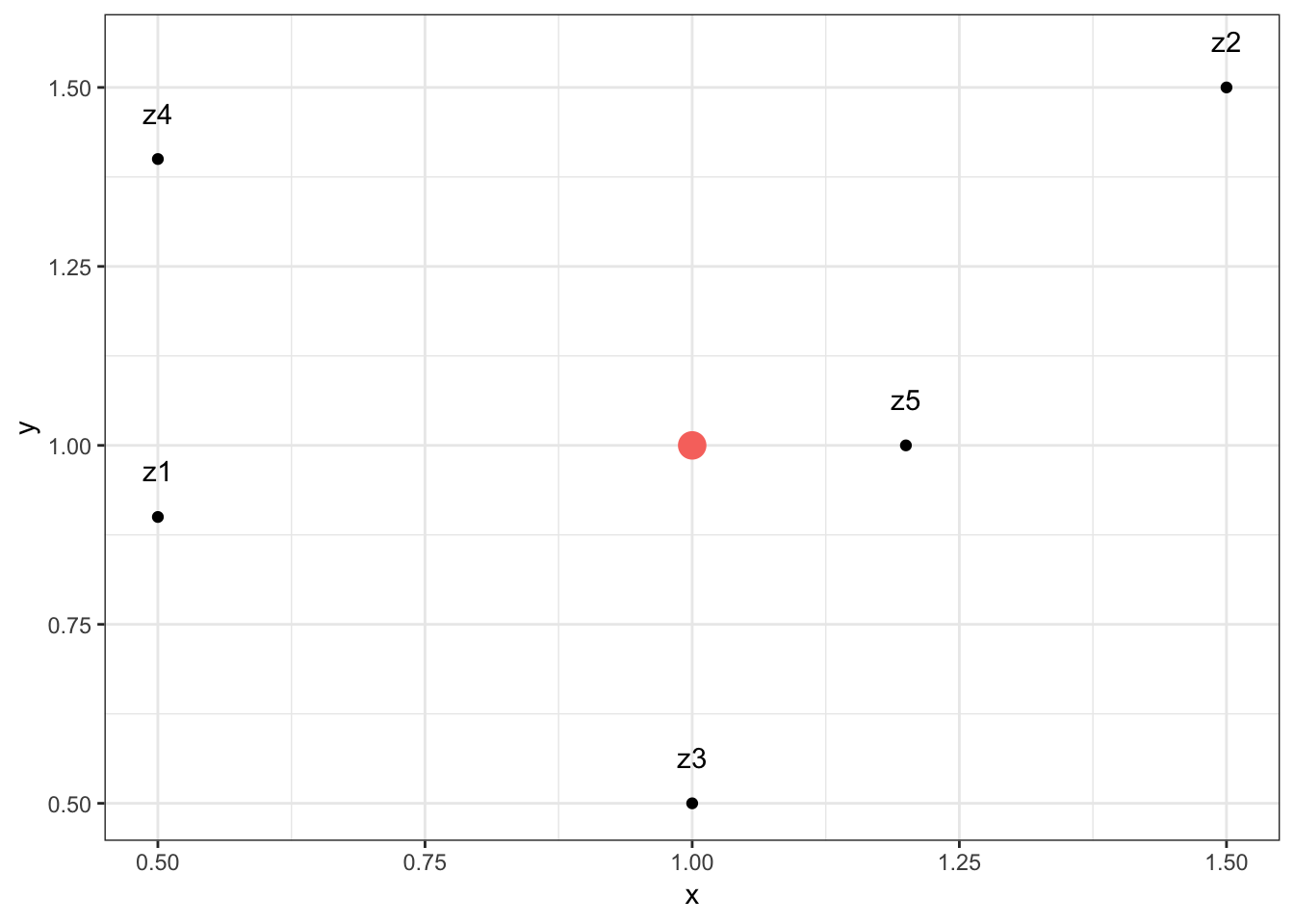In the inverse distance weighting (IDW) approach, also referred to as inverse distance-based weighted interpolation, the estimation of the value $$z$$ at location $$\mathbf x$$ is a weighted mean of nearby observations.

$\hat z(\mathbf x) = \frac{\sum_i^n w_iz_i}{\sum_i^nw_i}\text{,}$

where

$w_i = \vert \mathbf x-\mathbf x_i \vert^{-\beta}$ and where $$\beta \ge0$$ and $$\vert \cdot \vert$$ corresponds to the euclidean distance. The inverse distance power, $$\beta$$, determines the degree to which the nearer point(s) are preferred over more distant points. Typically $$\beta =1$$ or $$\beta =2$$ corresponding to an inverse or inverse squared relationship. The number of surrounding points, $$n$$, to be included decides whether a global or local weighting is applied. Both parameters $$\beta$$ and $$n$$ may be fine tuned by cross-validation, a technique we discuss in the subsequent sections in more detail. If the point $$\mathbf x$$ coincides with an observation location $$(\mathbf x= \mathbf x_i)$$, then the observed value, $$\mathbf x$$, is returned to avoid infinite weights.

Let us gain intuition on the IDW by applying it on a toy example. Given below are five points $$z_1$$, $$z_2$$, $$z_3$$, $$z_4$$, $$z_5$$ and one point $$z_0$$, which we want to predict, where $$z \in \mathbb R^2$$. The planar coordinates of each point $$z$$ are given in the columns x and y, and the actual value associated to each point is given in column z.

z0 <- c(1, 1, NA)
z1 <- c(0.5, 0.9, 1)
z2 <- c(1.5, 1.5, 3)
z3 <- c(1, 0.5, 5)
z4 <- c(0.5, 1.4, 7)
z5 <- c(1.2, 1, 7)
p <- as.data.frame(rbind(z0, z1, z2, z3, z4, z5))
colnames(p) <- c('x', 'y', 'z')
p
##      x   y  z
## z0 1.0 1.0 NA
## z1 0.5 0.9  1
## z2 1.5 1.5  3
## z3 1.0 0.5  5
## z4 0.5 1.4  7
## z5 1.2 1.0  7

We plot the points for a better understanding.

library(tidyverse)
p0 <- p[1,]
p1 <- p[2:nrow(p),]
ggplot(p1, aes(x = x, y = y, label = rownames(p1))) +
geom_point() +
geom_point(data = p0, aes(x = x, y = y,
label = rownames(p0), color = 'red',
cex = 5)) +
geom_text(vjust = 0, nudge_y = 0.05) +
theme_bw() +
theme(legend.position = "none")The euclidean distance, $$d$$, between two points $$(\mathbf u,\mathbf v)$$ in a plane $$(\mathbb R^2)$$ is given by

$d(\mathbf u,\mathbf v) = \sqrt{(u_1-v_1)^2 +(u_2-v_2)^2}$

Hence, we can compute $$w_i$$ as follows

$\left[ \begin{array}\\ w_1\\ w_2\\ ...\\ w_5 \end{array} \right] = \left[ \begin{array}\\ \vert \mathbf z-\mathbf z_1 \vert^{-\beta}\\ \vert \mathbf z-\mathbf z_2 \vert^{-\beta}\\ \cdots\\ \vert \mathbf z-\mathbf z_5 \vert^{-\beta} \end{array} \right]$

Consider $$\beta = 2$$ and $$n=5$$.

beta = 2
n = 5

Plugging in our toy data results in

$\left[ \begin{array}\\ w_1\\ w_2\\ w_3\\ w_4\\ w_5 \end{array} \right]= \left[ \begin{array}\\ \vert \mathbf z-\mathbf z_1 \vert^{-\beta}\\ \vert \mathbf z-\mathbf z_2 \vert^{-\beta}\\ \vert \mathbf z-\mathbf z_3 \vert^{-\beta}\\ \vert \mathbf z-\mathbf z_4 \vert^{-\beta}\\ \vert \mathbf z-\mathbf z_5 \vert^{-\beta} \end{array} \right] = \left[ \begin{array}\\ \sqrt{(1-0.5)^2 +(1-0.9)^2}^{- 2}\\ \sqrt{(1-1.5)^2 +(1-1.5)^2}^{- 2}\\ \sqrt{(1-1)^2 +(1-0.5)^2}^{- 2}\\ \sqrt{(1-0.5)^2 +(1-1.4)^2}^{- 2}\\ \sqrt{(1-1.2)^2 +(1-1)^2}^{- 2} \end{array} \right] \approx \left[ \begin{array}\\ 3.846\\ 2\\ 4\\ 2.439\\ 25 \end{array} \right]$

w <- sqrt((as.numeric(p0['x'])-p1['x'])^2 +
(as.numeric(p0['y'])-p1['y'])^2)^-beta
colnames(w) <- 'w'
round(w,3)
##         w
## z1  3.846
## z2  2.000
## z3  4.000
## z4  2.439
## z5 25.000

Hence,

$\sum_i^nw_i \approx 37.285$

round(sum(w),3)
##  37.285

Following that, we may write

$w_iz_i = \left[ \begin{array}\\ 3.846\\ 2\\ 4\\ 2.439\\ 25 \end{array} \right] \left[ \begin{array}\\ 1\\ 3\\ 5\\ 7\\ 7 \end{array} \right]$

resulting in

$\sum_i^n w_iz_i = 221.919$

round(sum(w*p1[,3]),3)
##  221.919

Plugging the numerator $$(\sum_i^n w_iz_i)$$ and the denominator $$(\sum_i^n w_i)$$ into the equation from above yields

$\hat z_0 = \frac{\sum_i^n w_iz_i}{\sum_i^nw_i} \approx \frac{221.919}{37.285} = 5.952$

hat.z0 <- round(sum(w*p1[,3])/ sum(w),3)
hat.z0
##  5.952

Hence, we conclude that given $$beta = 2$$ and $$n = 5$$, the value for location $$z_0\approx 5.952$$.

Note that usually interpolation is not done for one particular point in space but on a regular grid.